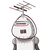# Q.10. A car is running at the speed of 72 Km/h. how much distancewill it cover in 22 minutes.On​

Q.10. A car is running at the speed of 72 Km/h. how much distance
will it cover in 22 minutes.
On​

### 1 thought on “Q.10. A car is running at the speed of 72 Km/h. how much distance<br />will it cover in 22 minutes.<br />On​”

1.### Given:

• Speed of a car = 72 km/h
• Time in which the car covers a particular distance = 22 minutes

### Tofind:

• Distance covered by the car

### ConceptUsed:

→ Formula to calculate distance :-

• Distance = S × T

where,

• S and T are speed and time respectively.

Unit conversion :-

• 1 min = 1/60 hr

To convert any value from min into hr, divide it by 60.

### Solution :

Firstly, convert the time from min into hr.

Time →

⠀⠀⠀⇒ Time = 22 min = 22/60 hr or 0.367 hr

Time = 22/60 hr or 0.367 hr

Distance covered by the car :-

⠀⠀⠀⇒ Distance = S × T

⠀⠀⠀⇒ Distance = 72 × 22/60

⠀⠀⠀⇒ Distance = 26.4

Distance covered by the car in 22 minutes or 0.367 hr = 26.4 km

⠀⠀⠀⠀━━━━━━━━━━

### Know MorE :

• Distance is the path travelled by a body in a particular time.
• Speed is the rate at which a body covers a particular distance.
• Speed = Distance ÷ Time
• Time = Distance ÷ Speed